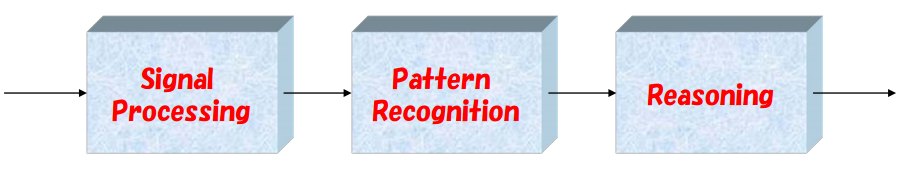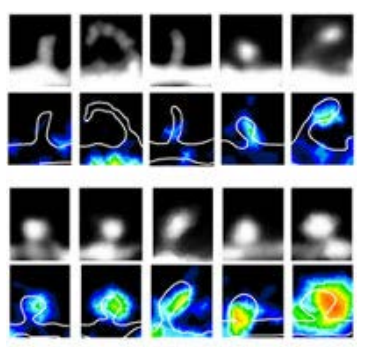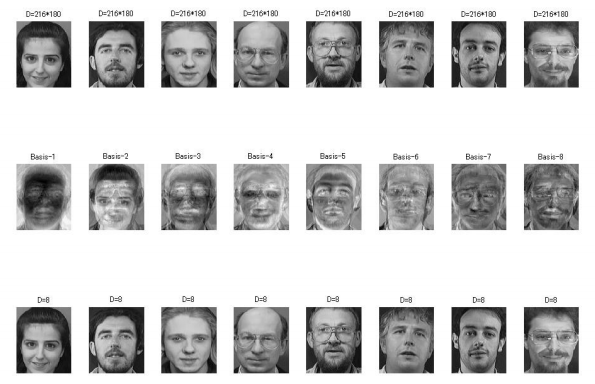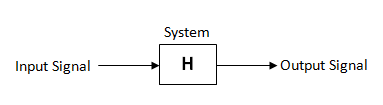# Introduction to Signals And Systems

## What is a signal ?

Any physical phenomenon that conveys or carries some information can be called a signal. A signal is anything you can see, hear, observe or measure using some machine. For examples: Speech, audio, light, radio, TV, radar, supersonic, temperature, ECG, EEG, etc .

Usually, the information carried by a signal will be a function of an independent variable. The independent variable can be time, spatial coordinates, intensity of colors, pressure, temperature, etc.

The most popular independent variable in signal is time and it is represented by  the letter “t”.

The value of signal at any specified value of independent variable is called  its amplitude. The sketch of the amplitude of a signal as a function of independent variable is called its waveform.

Mathematically, any signal can be represented as a function of one or more independent variables. Therefore , a signal is defined as any physical quantity that varies with one or more independent variables.

Examples:

x1(t) = 0.5 t               (varies linearly with t)

x2(t) = 0.8 t2                ( varies quadratically with t )

x(p , q) = 0.3 p + 0.6 q + 0.2 t2             ( function of two independent variables p and q )

### Signal Classification

The signal can be classified in number of ways. Some way of classifying the signals are,

• Depending on the number of sources for the signals:
1. One channel signals
2. Multi channel signals
• Depending on the number of dependent variables:
1. One dimensional signals
2. Multidimensional signals
• Depending on whether the dependent variable is continuous or discrete:
1. Analog or continuous signals
2. Discrete signals

#### One Channel Signals

Signals that are generated by single source are called one channel signals.

Examples: Record of room temperature with respect to time, the audio output of a mono speaker, etc.

#### Multi Channel Signals

Signals that are generated by multiple sources are called multi channel signals.

Examples: The audio output of two stereo speakers (two-channel signal), the record of ECG  at eight different places in a human body (eight channel signal)

#### One Dimensional Signals

A signal which is a function of single independent variable is called one dimensional signal.

Examples: music, speech, heart beat, etc.

#### Multidimensional Signals

A signal which is a function of two or more independent variables is called multidimensional signal.

Examples: A photograph is an example of two dimensional signal, the motion picture of a black and white TV is an example of three dimensional signal.

#### Analog or Continuous Signals

When a signal is defined continuously for any value of independent variable, it is called analog or continuous signal.

#### Discrete Signals

When a signal is defined for discrete intervals of independent variables, it is called discrete signal .

Most of the discrete signals are either sampled version of analog signals or output of digital systems.

## What is signal processing ?

The purpose of signal processing is to  extract useful information from the signal and to make correct decisions.

For this purpose, we have

1.  Signal enhancement
2.  Signal restoration
3.  Signal reconstruction
4.  Signal synthesis
5.  Signal estimation and prediction

After signal processing, we can extract more important information, and recognize, aware, and make decisions.### Signal Enhancement

Example of signal enhancement are :

1. Signal amplification in radio, TV, human ears
2. Image enhancement in image processing### Signal Restoration

Signal restoration is required to restore a signal corrupted by noise or useless background.

#### Applications:

• Tuning in radio and TV
• Noise cleaning
• Pick up the voice of a certain person in a partyFig.3: Restoration of face images

### Signal Reconstruction

Signal reconstruction means to reconstruct a signal from different measurement such as

•  Computerized tomogram (CT)
•  Synthetic aperture radar (SAR) imaging
• Reconstruct 3D images from different 2D views

### Signal Synthesis

Signal synthesis is nothing but to find a system that can generate the desired signal. For example:

• Speech synthesis
• 3D sound synthesis
• Make a virtual world in computer

### Signal Estimation

Signal estimation, estimate the future values from the observed data.Examples of signal estimation are:

• Stock prediction
• Electrical Demand prediction
• Image compression
• Adaptive signal processing

## System

Any process that exhibits cause and effect relation can be called as a system.

A system will have an input signal and an output signal.

The output signal will be a processed version of the input signal.

A system is either interconnection of hardware devices or software/ algorithms .

A system is denoted by letter H. The diagrammatic representation of  a system is shown below:The operation performed by a system on input signal to produce output signal can be expressed as :

Output = H[Input]

where H denotes the system operation or system operator

The system can be classified in many ways. We will discuss the classification of systems in our next article.